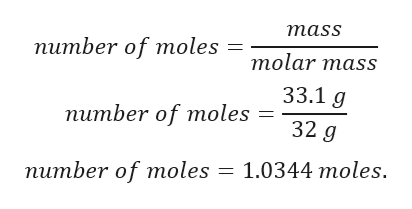# A sample of oxygen gas has a mass of 33.1 grams.How many O2 molecules are there in the sample?

Question

A sample of oxygen gas has a mass of 33.1 grams.

How many Omolecules are there in the sample?

check_circle

Step 1

Here, we have to calculate number of molecules present in 33.1 g of oxygen.

Step 2

Given:

Mass of oxygen = 33.1 g.

Molar mass of oxygen = 32 g.

Step 3

Calculation for number o...help_outlineImage Transcriptionclosemass number of moles molar mass 33.1 g number of moles 32 g number of moles 1.0344 moles. fullscreen

### Want to see the full answer?

See Solution

#### Want to see this answer and more?

Solutions are written by subject experts who are available 24/7. Questions are typically answered within 1 hour.*

See Solution
*Response times may vary by subject and question.
Tagged in

### Chemistry